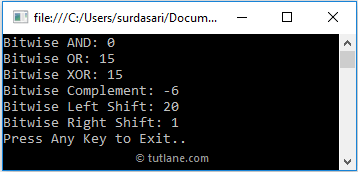# C# Bitwise Operators with Examples

In c#, Bitwise Operators will work on bits, and these are useful to perform bit by bit operations such as Bitwise AND (&), Bitwise OR (|), Bitwise Exclusive OR (^), etc. on operands. We can perform bit-level operations on Boolean and integer data.

For example, we have integer variables a = 10, b = 20, and the binary format of these variables will be shown below.

a = 10 (00001010)
b = 20 (00010100)

When we apply the Bitwise OR (|) operator on these parameters, we will get the result shown below.

00001010
00010100
-----------
00011110 = 30 (Decimal)

The following table lists the different types of operators available in c# bitwise operators.

OperatorNameDescriptionExample (a = 0, b = 1)
& Bitwise AND It compares each bit of the first operand with the corresponding bit of its second operand. If both bits are 1, then the result bit will be 1; otherwise, the result will be 0. a & b (0)
| Bitwise OR It compares each bit of the first operand with the corresponding bit of its second operand. If either of the bit is 1, then the result bit will be 1; otherwise, the result will be 0. a | b (1)
^ Bitwise Exclusive OR (XOR) It compares each bit of the first operand with the corresponding bit of its second operand. If one bit is 0 and the other bit is 1, then the result bit will be 1; otherwise, the result will be 0. a ^ b (1)
~ Bitwise Complement It operates on only one operand, and it will invert each bit of operand. It will change bit 1 to 0 and vice versa. ~(a) (1)
<< Bitwise Left Shift) It shifts the number to the left based on the specified number of bits. The zeroes will be added to the least significant bits. b << 2 (100)
>> Bitwise Right Shift It shifts the number to the right based on the specified number of bits. The zeroes will be added to the least significant bits. b >> 2 (001)

## C# Bitwise Operators Example

Following is the example of using the Bitwise Operators in the c# programming language.

using System;

namespace Tutlane
{
class Program
{
static void Main(string[] args)
{
int x = 5, y = 10, result;
result = x & y;
Console.WriteLine("Bitwise AND: " + result);
result = x | y;
Console.WriteLine("Bitwise OR: " + result);
result = x ^ y;
Console.WriteLine("Bitwise XOR: " + result);
result = ~x;
Console.WriteLine("Bitwise Complement: " + result);
result = x << 2;
Console.WriteLine("Bitwise Left Shift: " + result);
result = x >> 2;
Console.WriteLine("Bitwise Right Shift: " + result);
Console.WriteLine("Press Enter Key to Exit..");
}
}
}

If you observe the above code, we used different bitwise operators (&, |, ^, ~, <<, >>) to perform different operations on defined operands.

## Output of C# Bitwise Operators Example

When we execute the above c# program, we will get the result as shown below.This is how we can use bitwise operators in the c# programming language to perform bit by bit operations on defined operands based on our requirements.